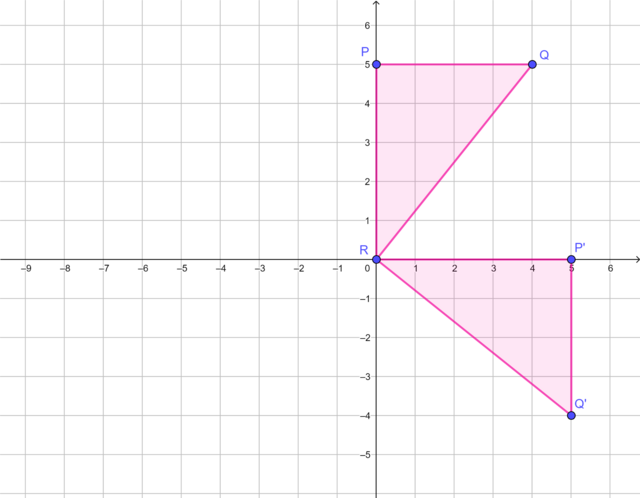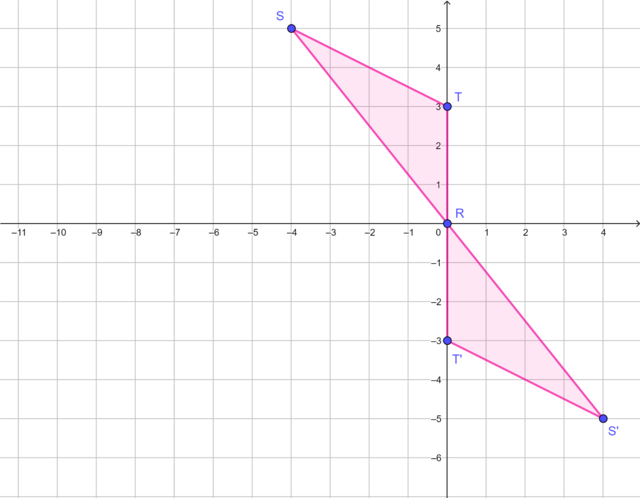Home Practice
For learners and parents For teachers and schools
Textbooks
Full catalogue
Pricing SupportLog in

We think you are located in United States. Is this correct?

# End of chapter exercises

Exercise 24.9 End of chapter exercises

Complete the following on the Cartesian plane:

1. Draw $$\triangle KLM$$ with vertices $$K(-5; 0)$$, $$L(-5; 3)$$ and $$M(0; 3)$$.

2. Draw $$\triangle K'L'M'$$, the image of $$\triangle KLM$$ rotated by $$180°$$ in a clockwise direction about the origin.

1.2.Draw the following on the Cartesian plane:

1. Plot $$\triangle ABC$$ with vertices $$A(-2; 0)$$, $$B(0; 5)$$ and $$C(0; 0)$$.

2. Draw $$\triangle A'B'C'$$, the image of $$\triangle ABC$$ rotated by $$90°$$ in an anti-clockwise direction about the origin.

1.2.Describe the rotation transformation.$$\triangle P'Q'R'$$ is the image of $$\triangle PQR$$ rotated by $$90°$$ in a clockwise direction about the origin.$$\triangle R'S'T'$$ is the image of $$\triangle RST$$ rotated by $$180°$$ in a clockwise (or anti-clockwise) direction about the origin.